2019-07-16 09:48:07 weixin_43612049 阅读数 185
• ###### 教你玩转ppt

教你玩转ppt

24139 人正在学习 去看看 刘青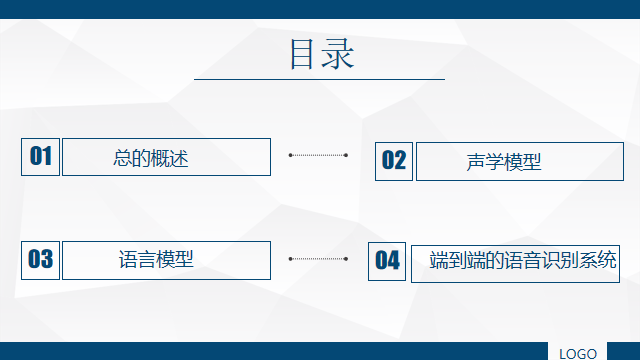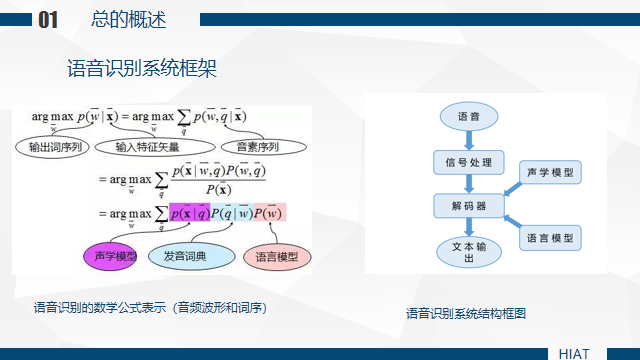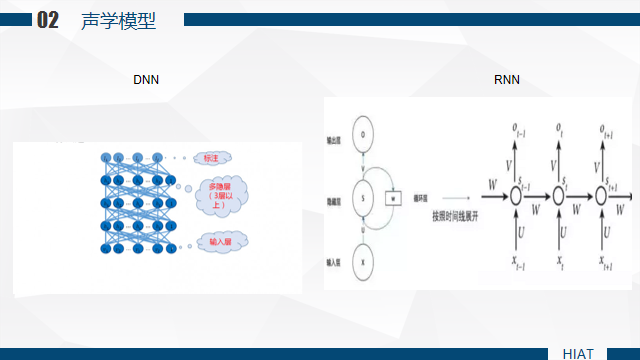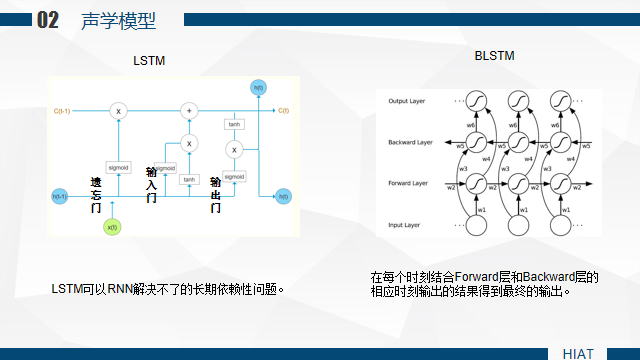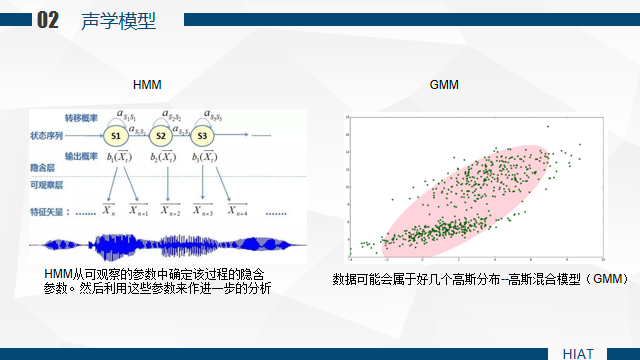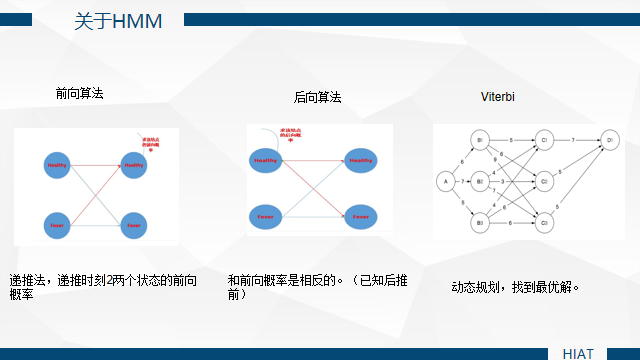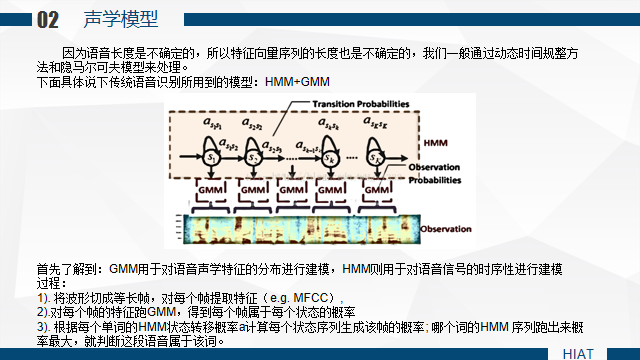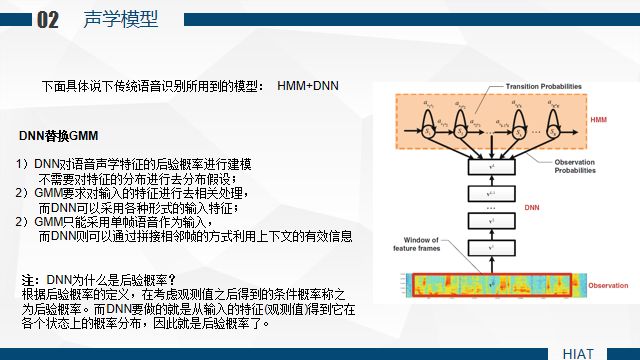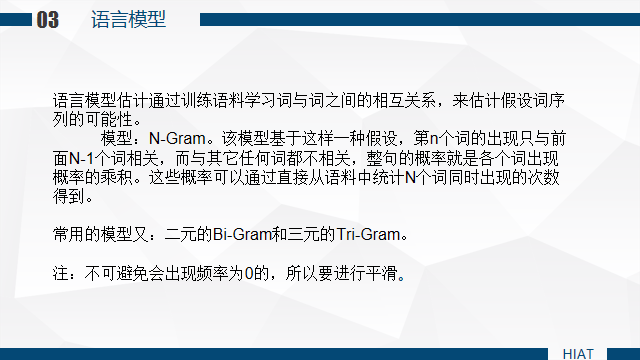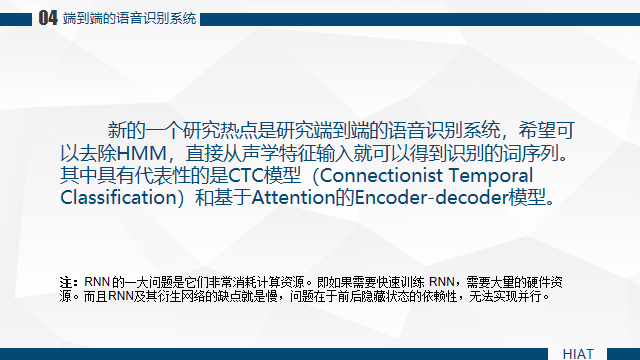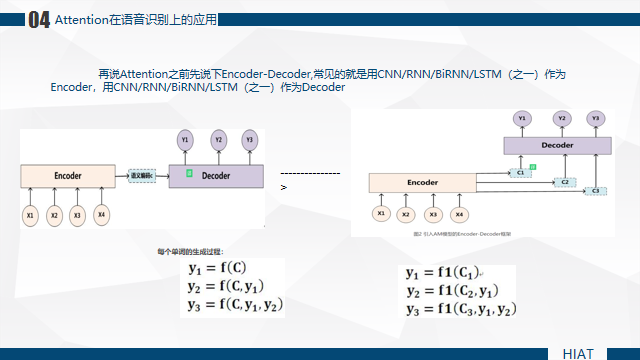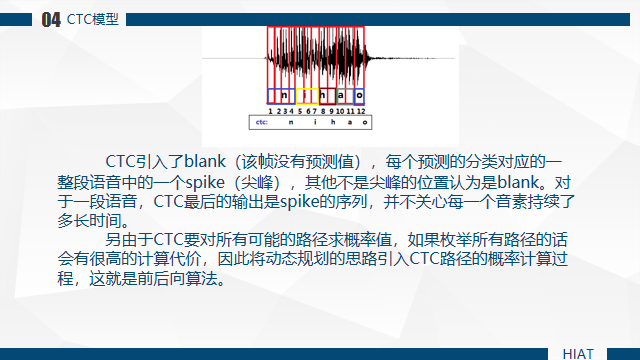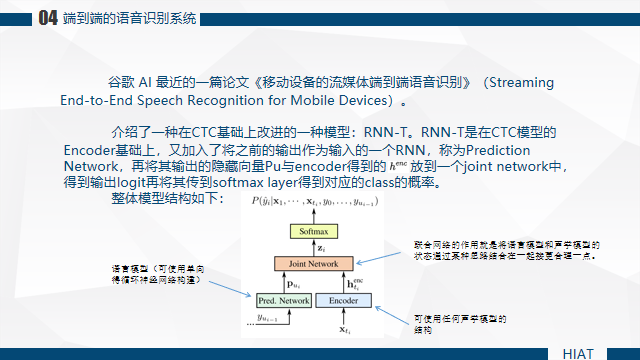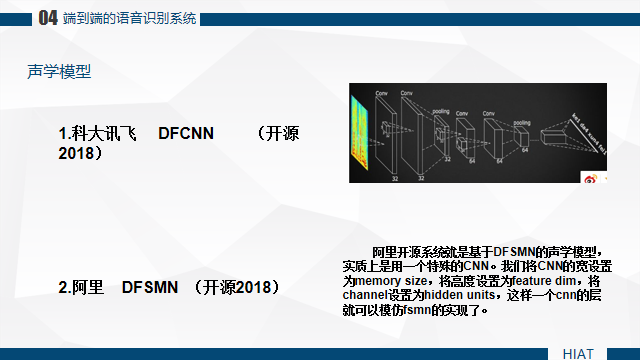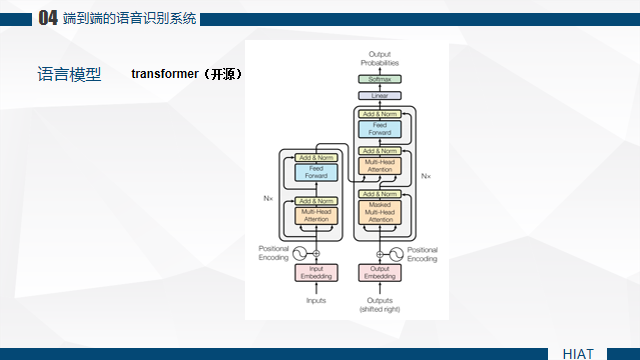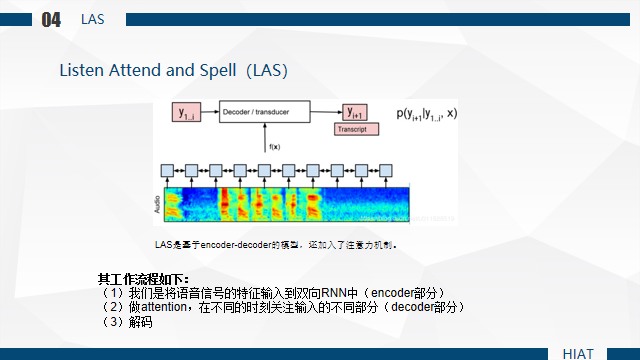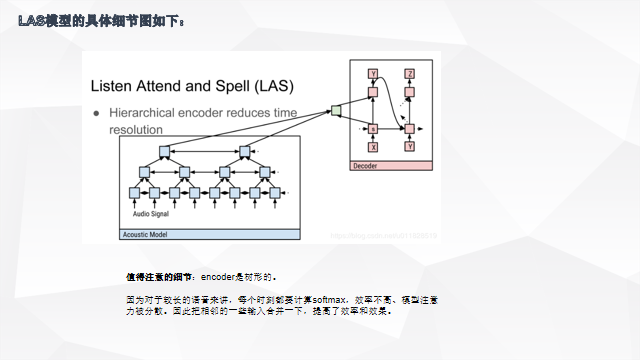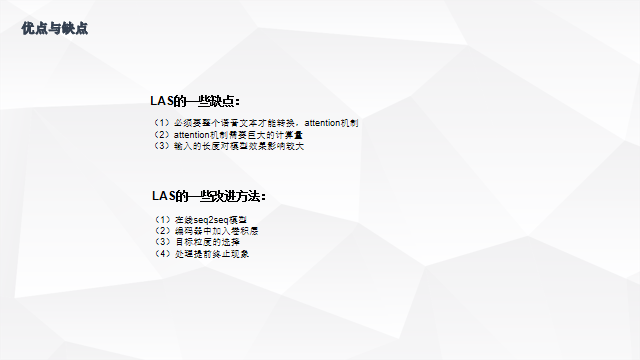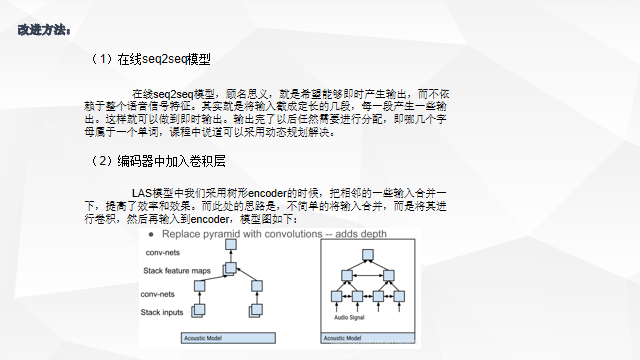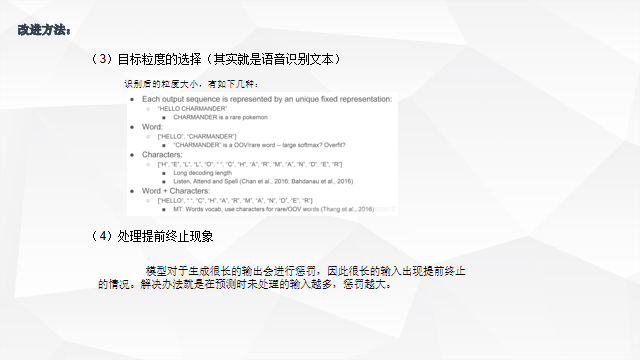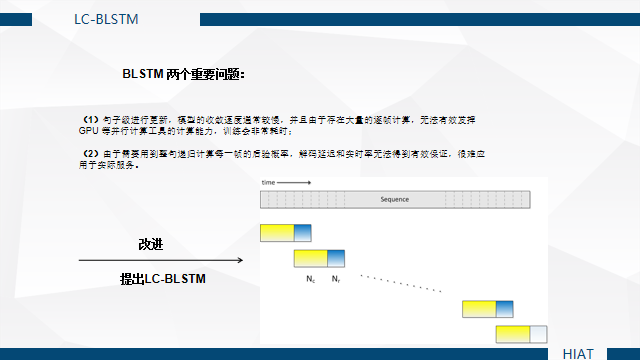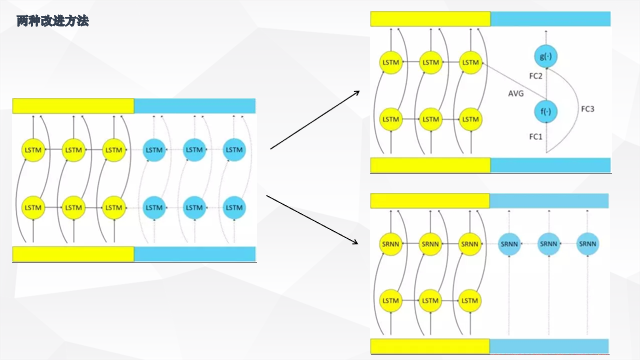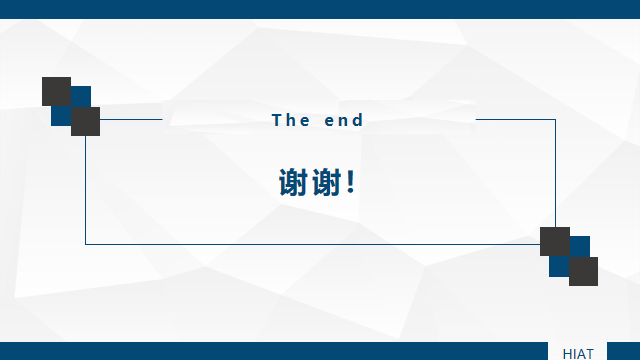2018-12-01 22:57:29 Tonywu2018 阅读数 2168
• ###### 教你玩转ppt

教你玩转ppt

24139 人正在学习 去看看 刘青

1.背景

2.孤立词识别

2.1 特征提取

2.2 动态弯算法

2.3 GMM(Gaussian mixture model)

2.4 HMM(Hidden markov model)

2.5 EM训练算法

2.6 语音识别基本方程

3.连续语音识别

3.1 语言模型

3.2 大词汇量

3.3 语音识别系统结构

3.4 评价指标：WER

4. 潘多拉魔盒

4.1 上下文有关模型

4.2 区分式训练

4.3 说话人适应

4.4 二次打分

5.结语

# 1.背景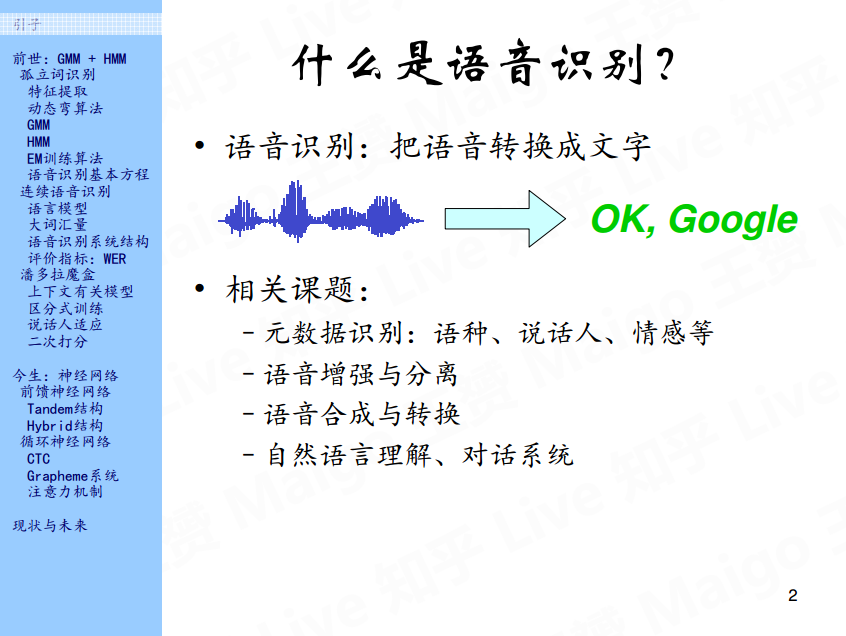# 2.孤立词识别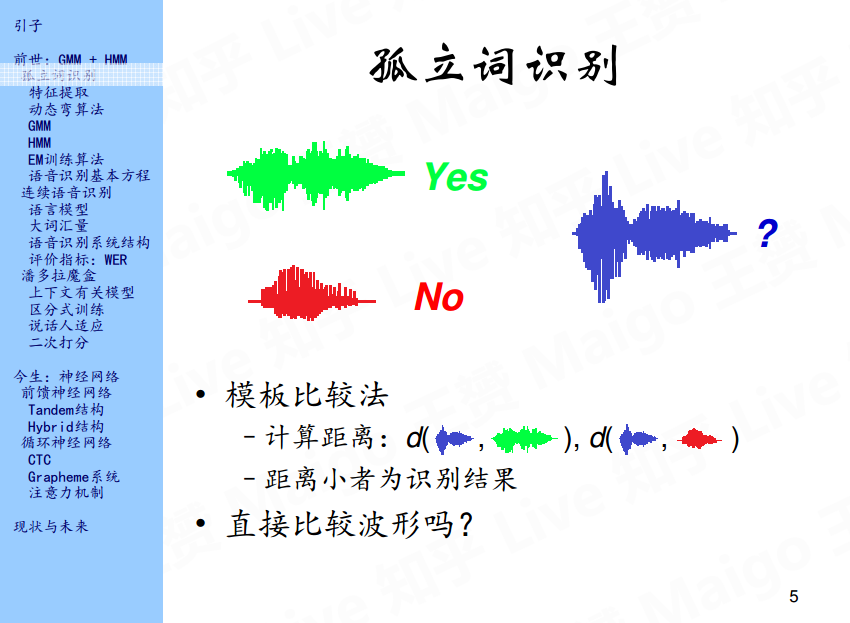## 2.1 特征提取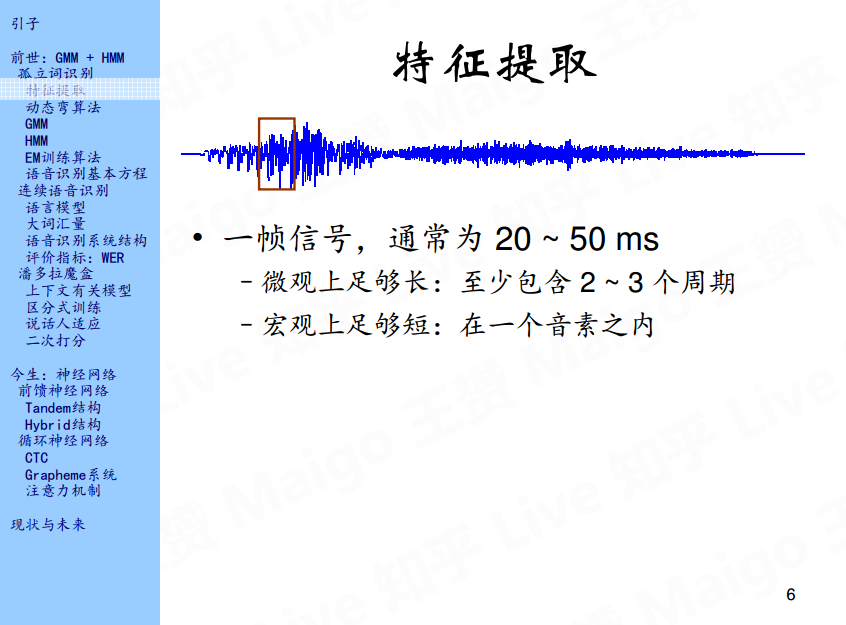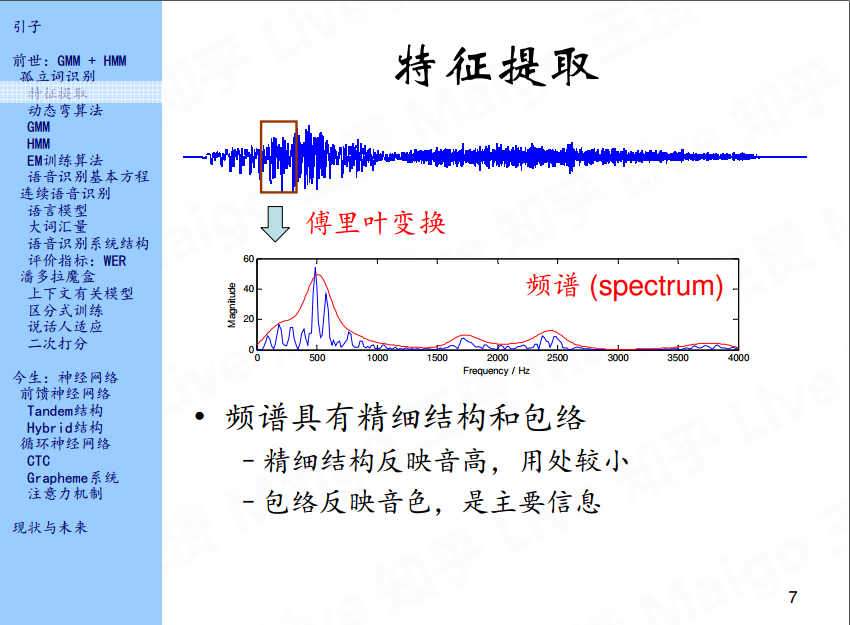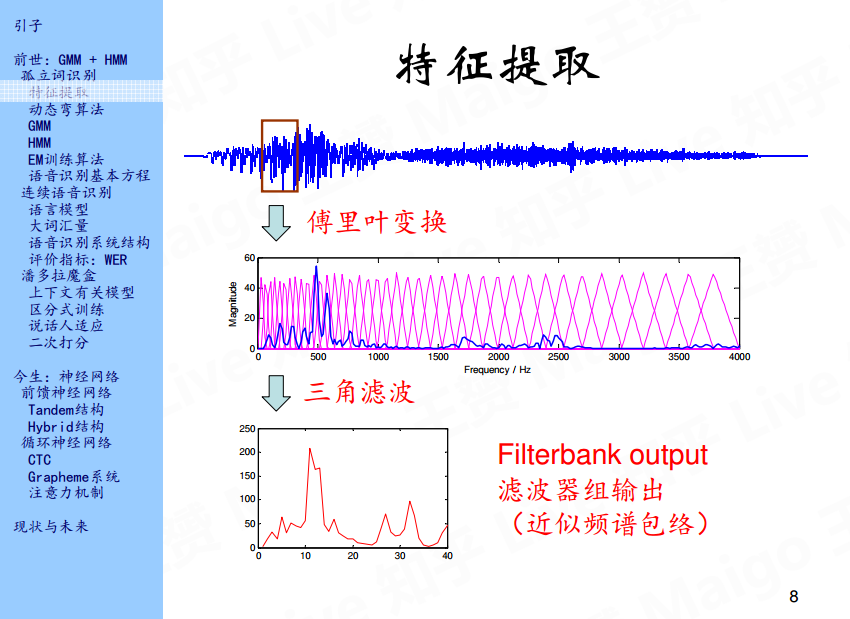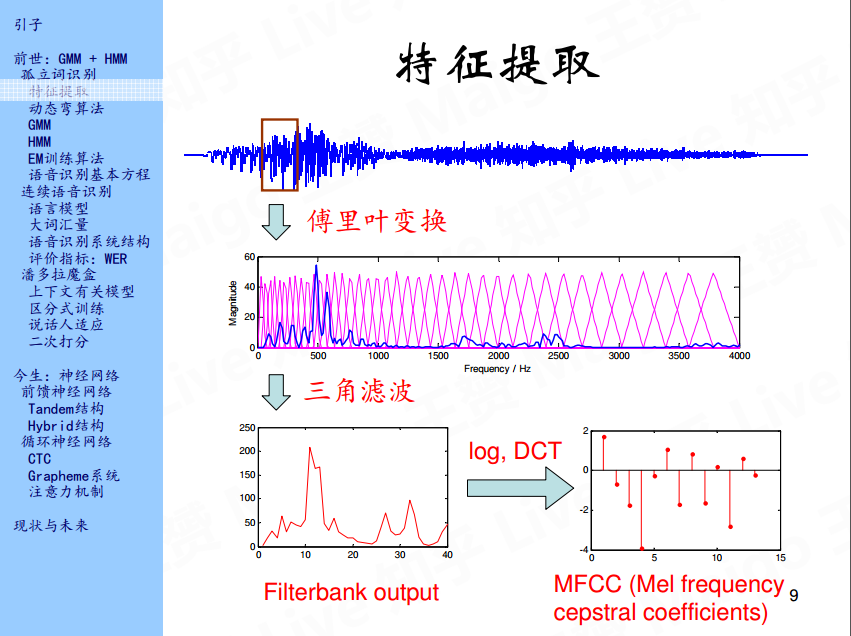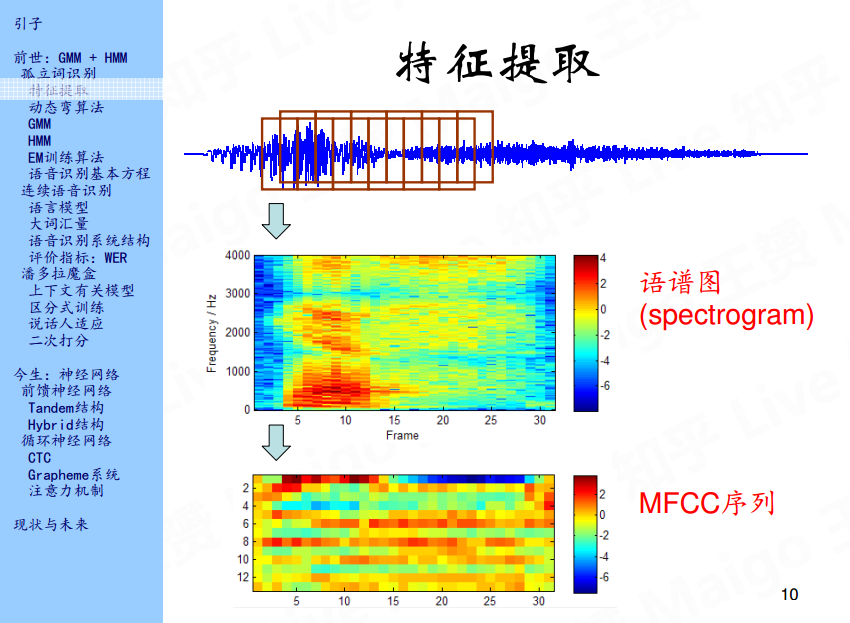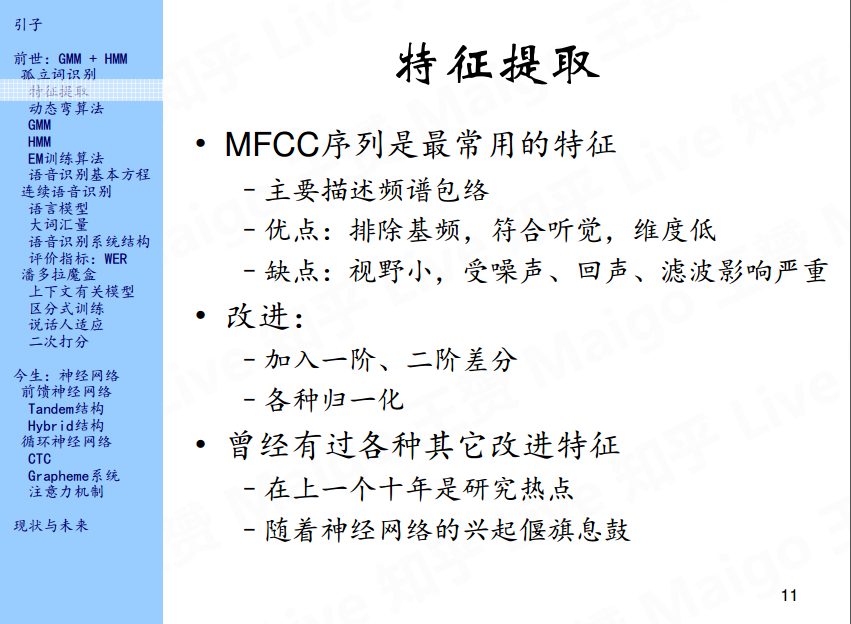## 2.2 动态弯算法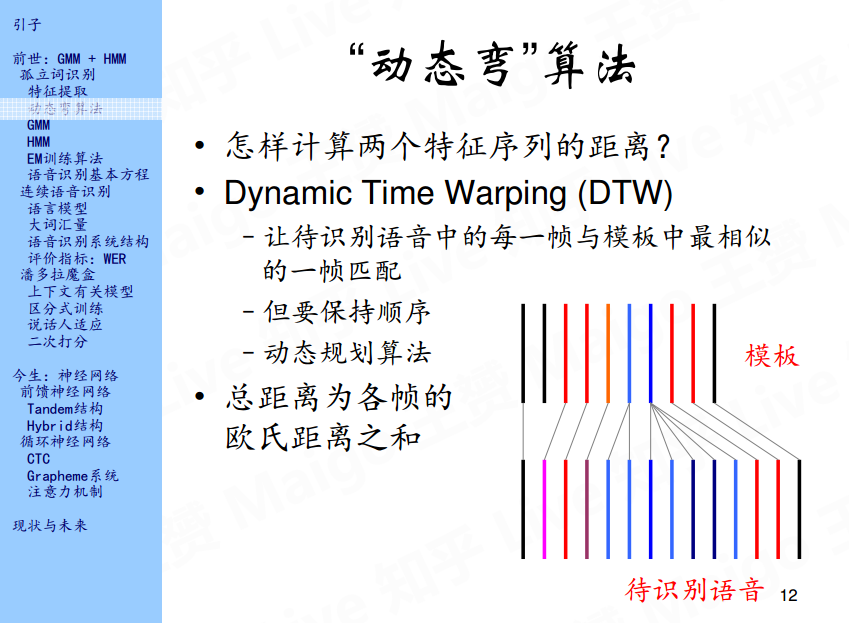## 2.3 GMM(Gaussian mixture model)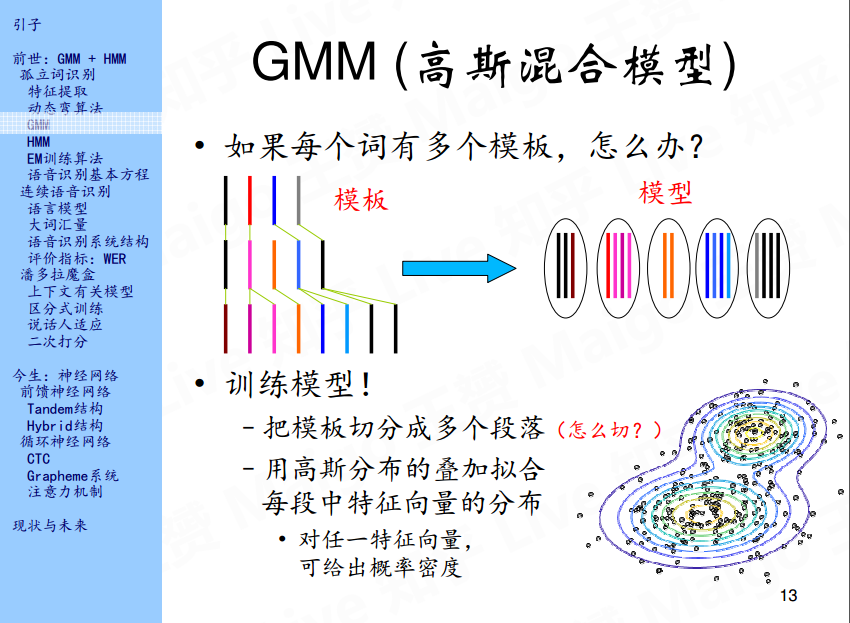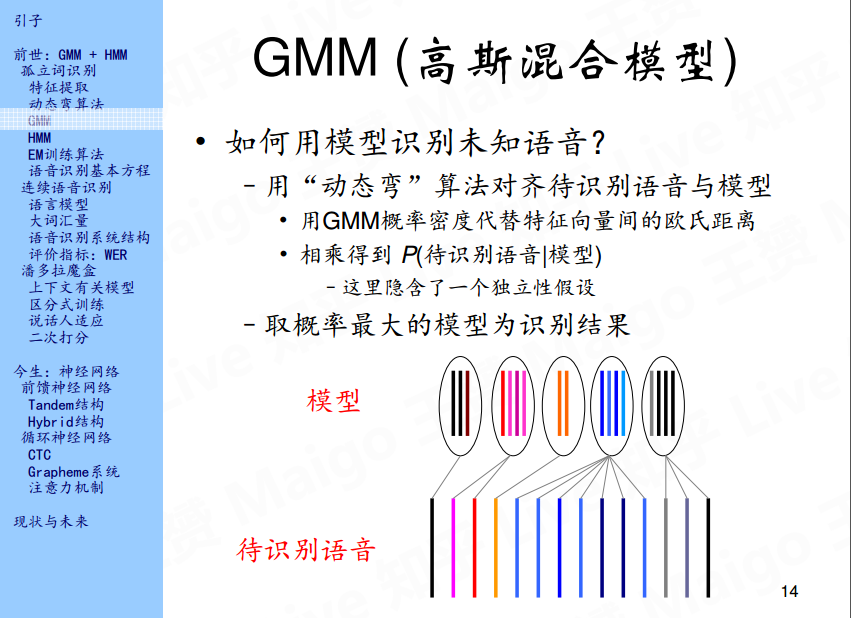## 2.4 HMM(Hidden markov model)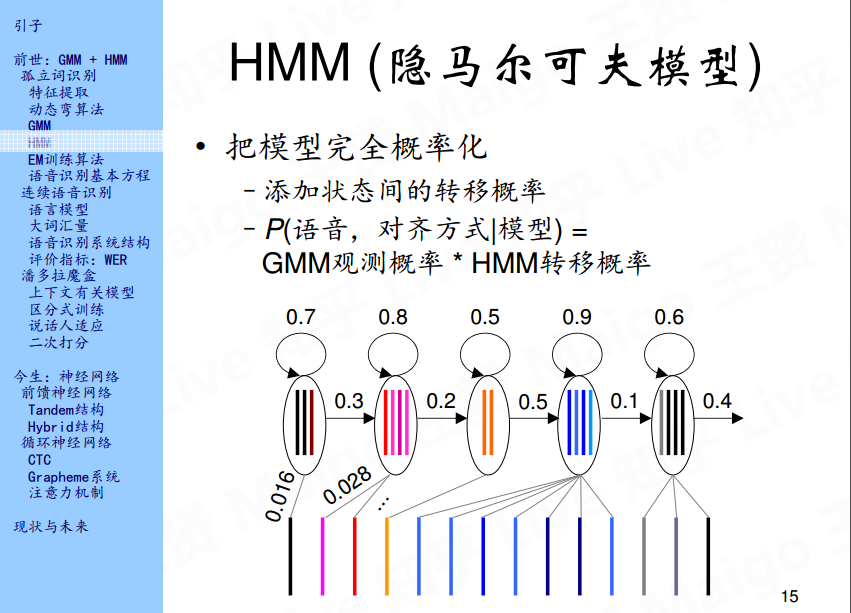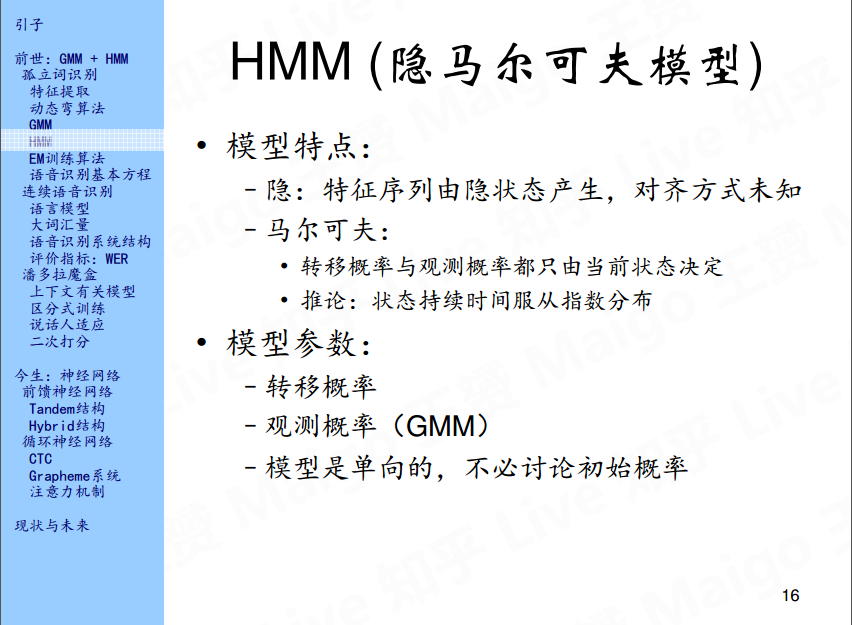HMM主要有两个特点，第一个就是特征序列由隐状态产生，隐状态也就是上面说到的五个状态，为什么称之为隐状态呢，是因为每帧特征向量和状态之间的对齐方式是未知的；另一个特点就是马尔可夫性，这里就不多说了。有关GMM，HMM，包括下面将要说的EM的相关知识，我推荐大家可以看一下悉尼科技大学的徐亦达老师在优酷的一个自频道，里面详细的讲解了概率统计的一些知识，并且每一个概念都有详细的公式推导，值得一看，教学视频见参考文献3。说完模型的特点，接下来说一下模型的参数，实际上HMM是有三个参数的：转移概率，观测概率以及初始概率。但是上面示例中的HMM是单向的，所以初始状态默认为第一个状态，所以，模型的参数就变成了两个：转移概率和观测概率。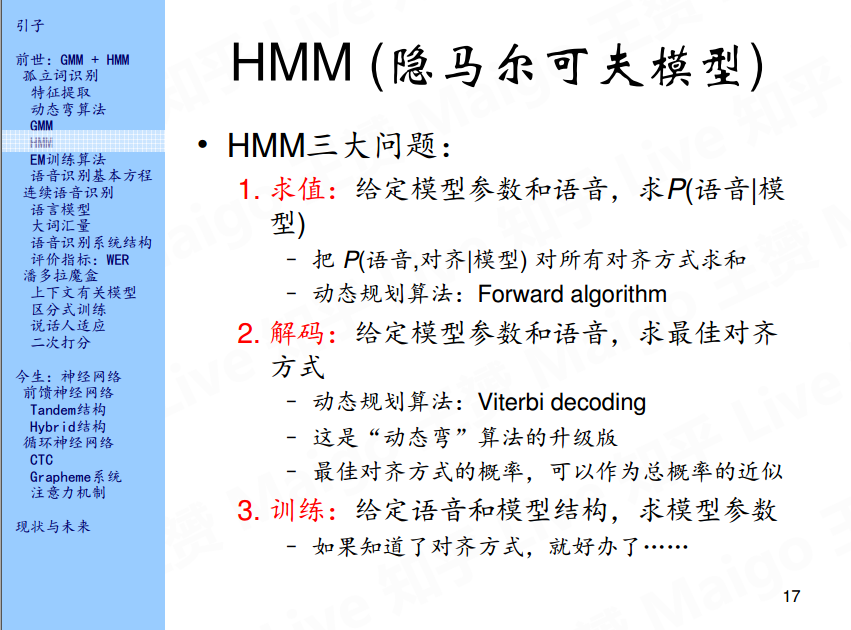## 2.5 EM训练算法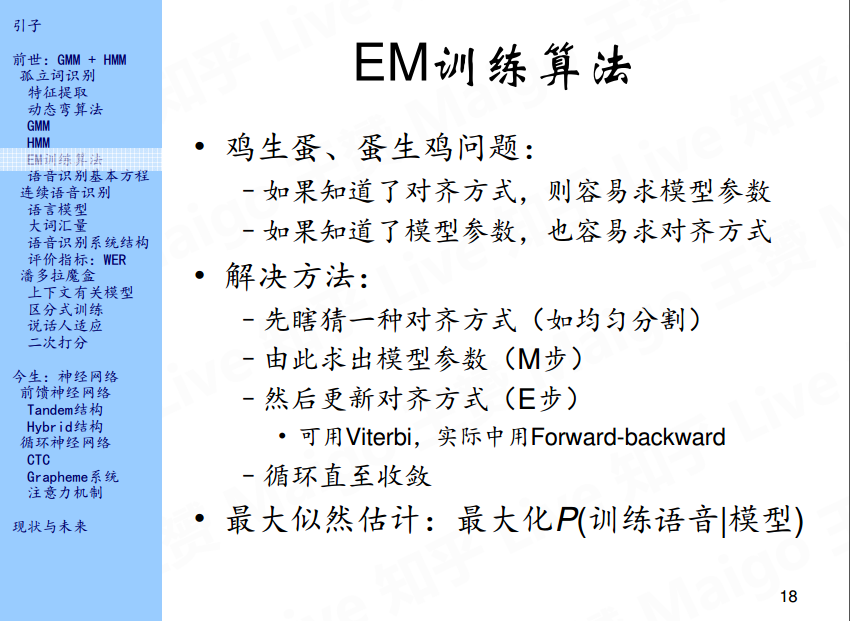## 2.6 语音识别基本方程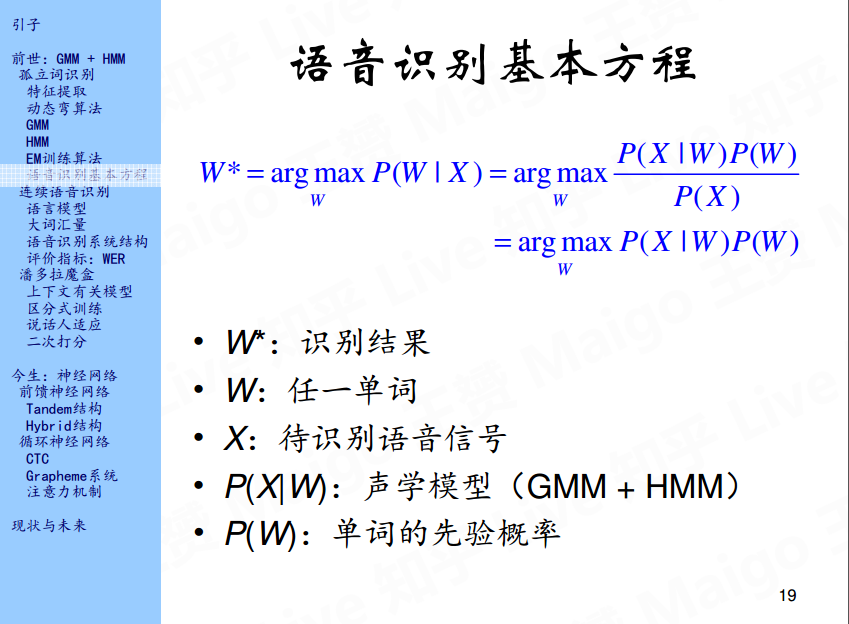# 3.连续语音识别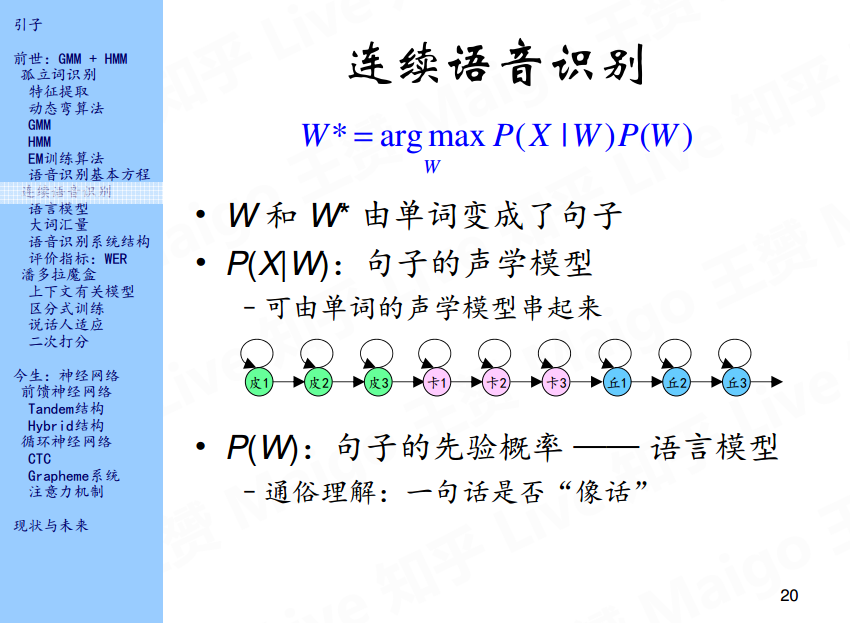## 3.1 语言模型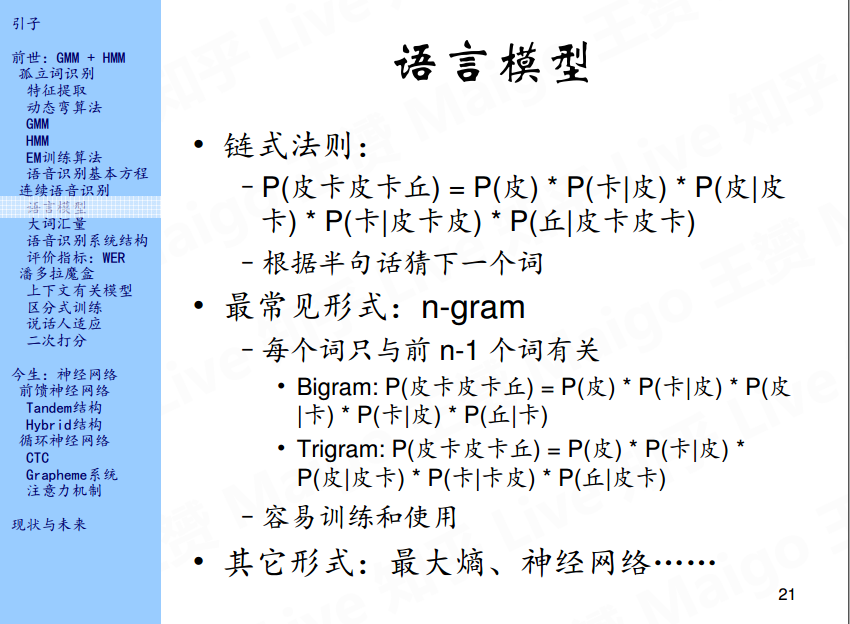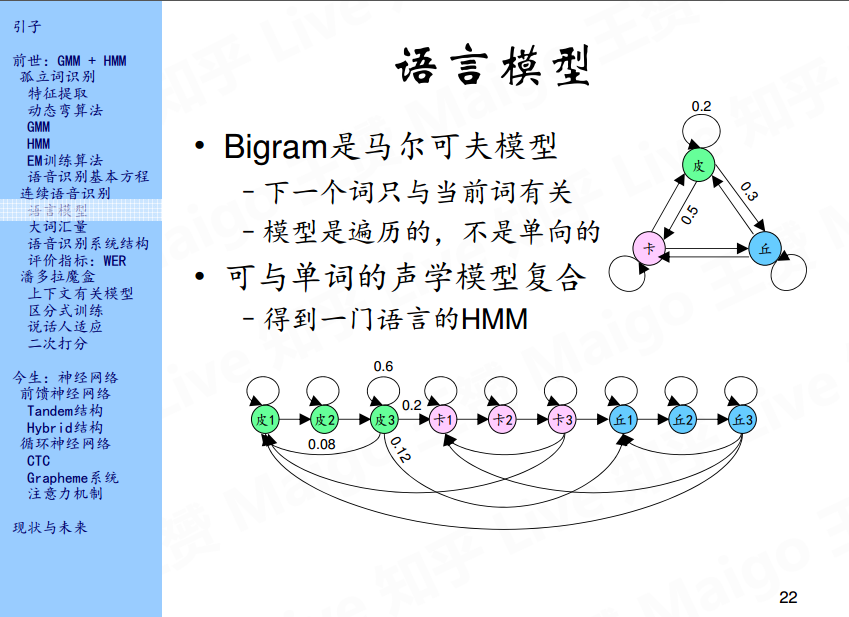## 3.2 大词汇量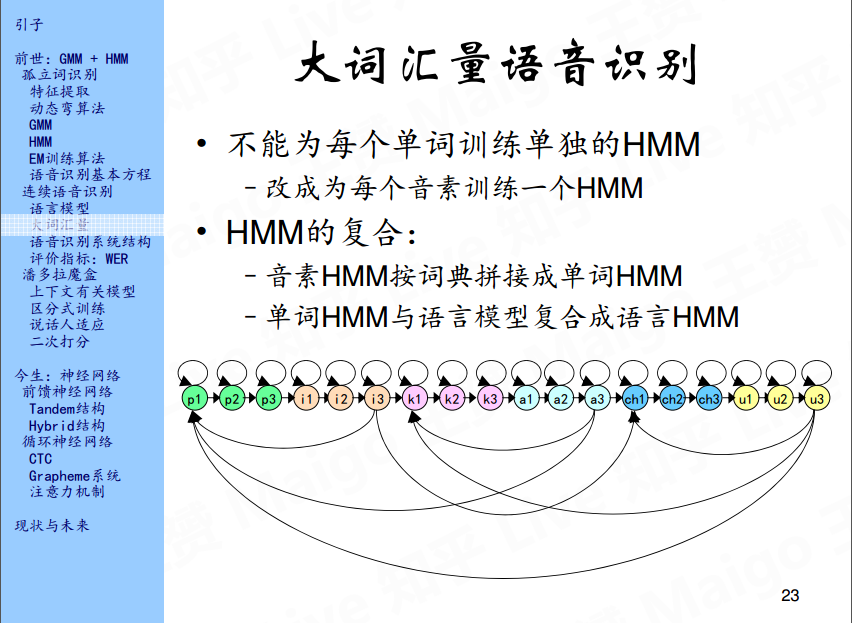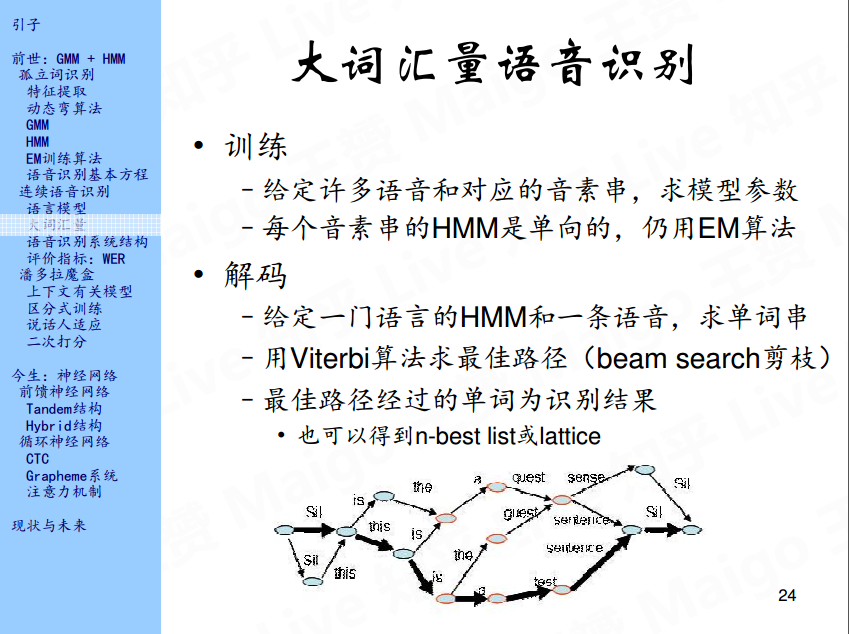## 3.3 语音识别系统结构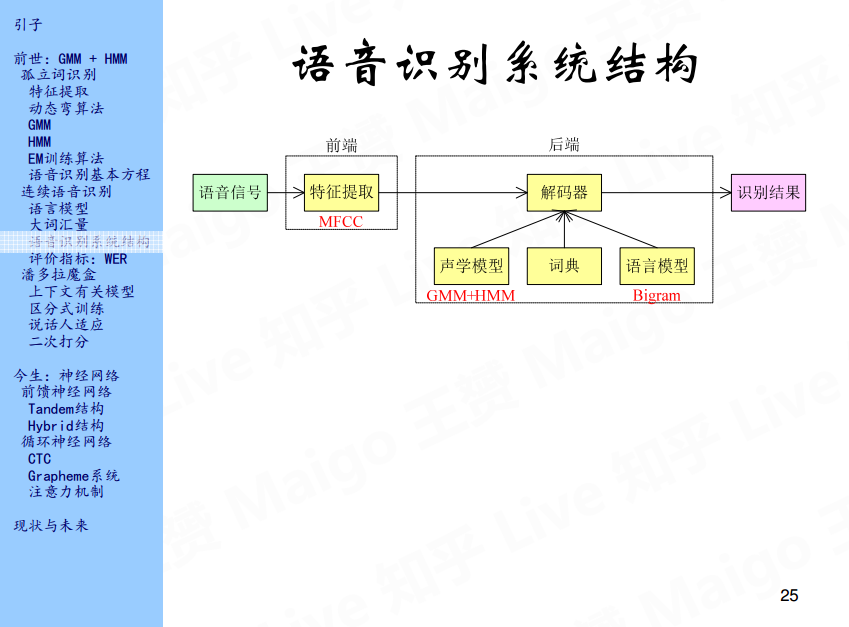## 3.4 评价指标：WER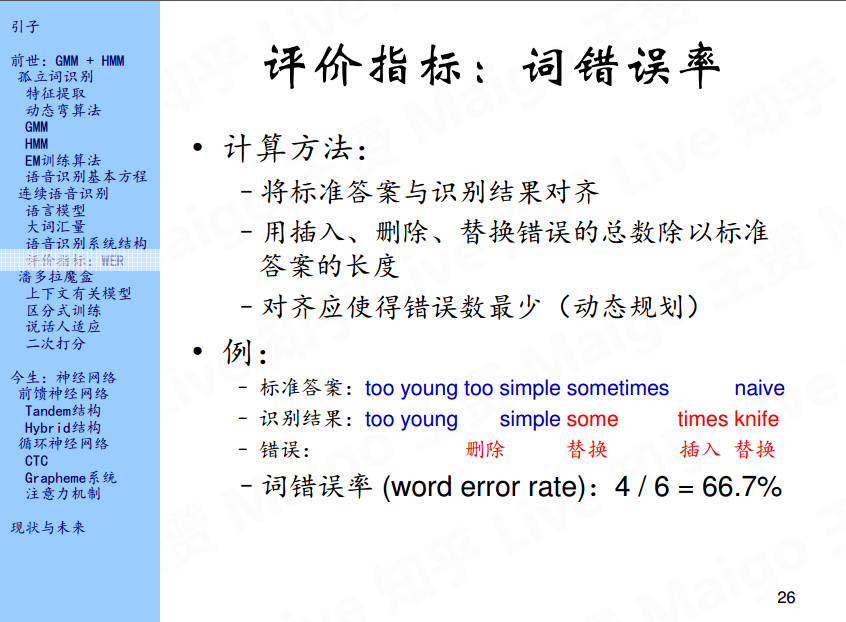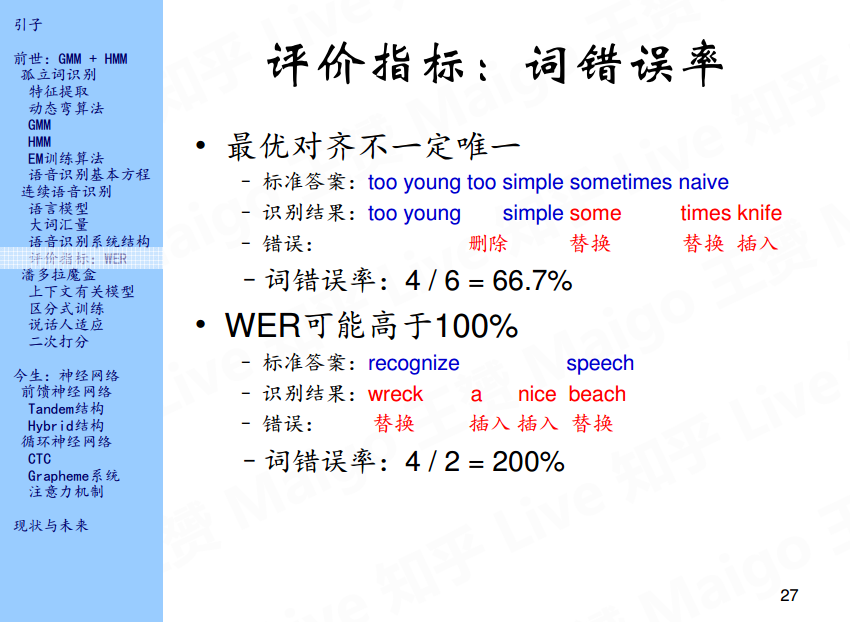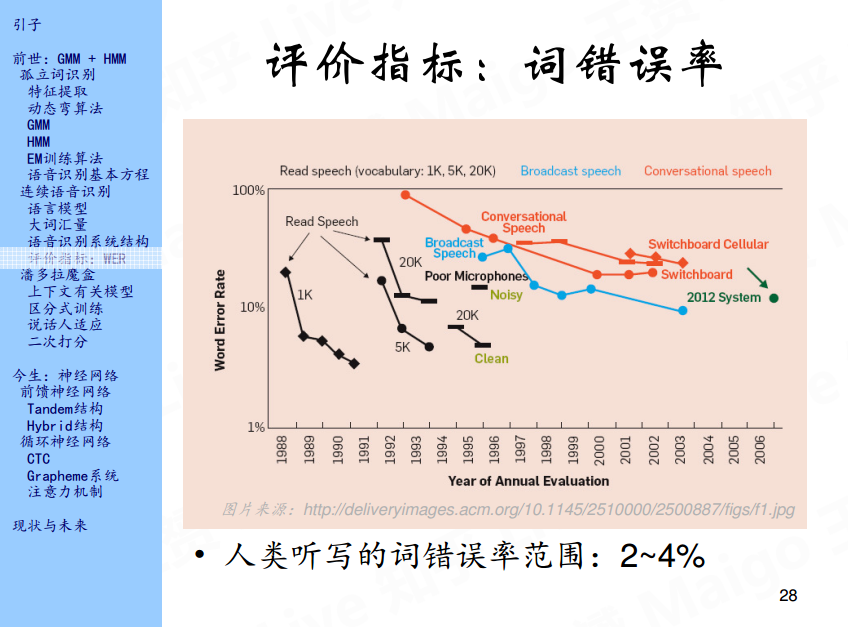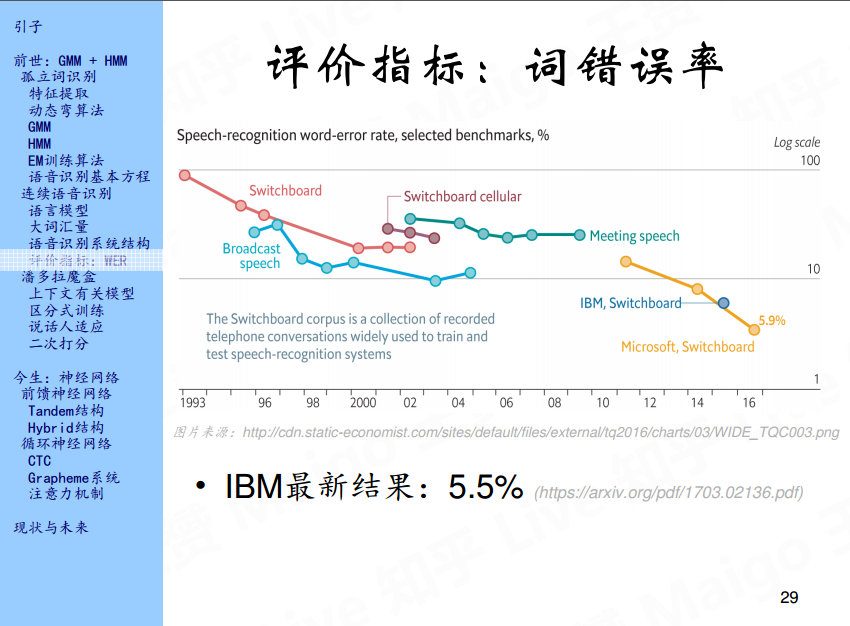# 4. 潘多拉魔盒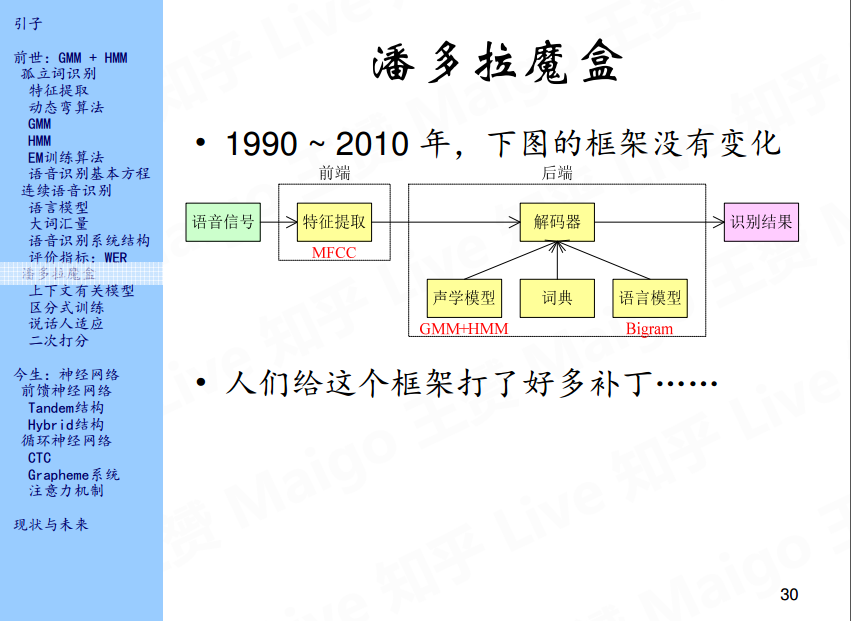## 4.1 上下文有关模型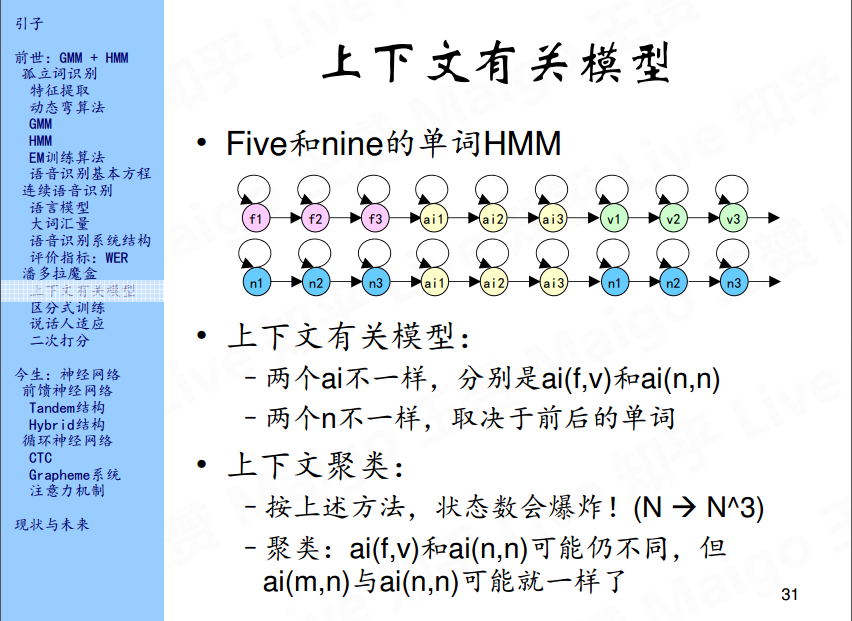## 4.2 区分式训练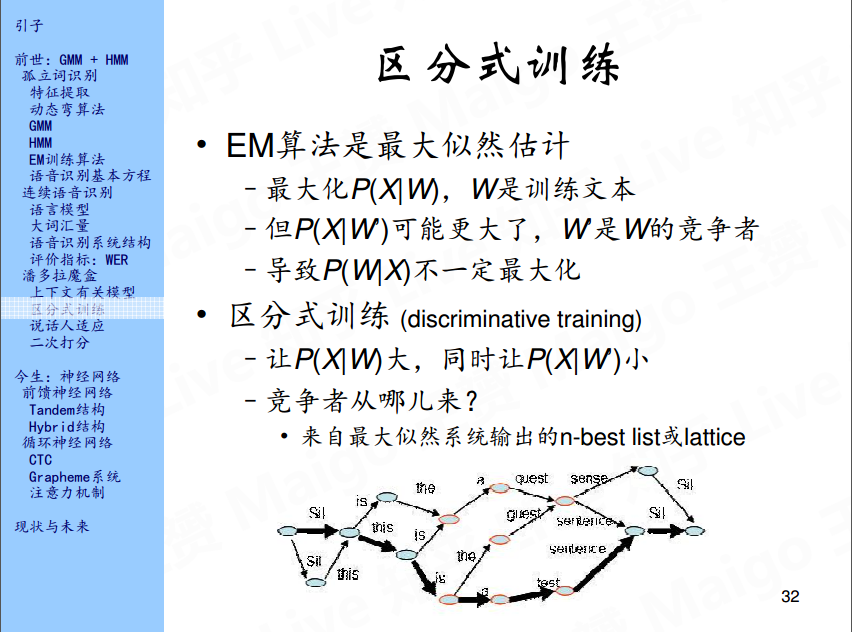## 4.3 说话人适应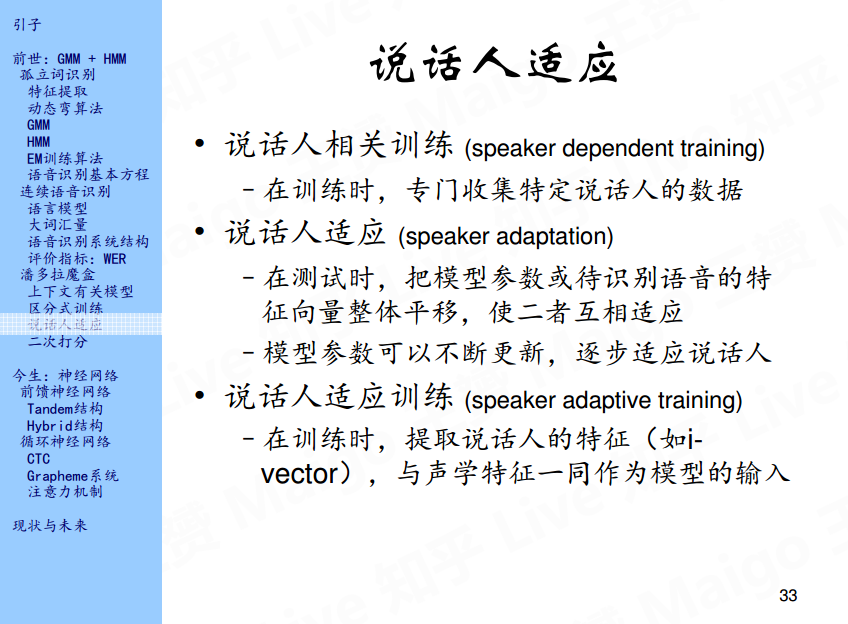## 4.4 二次打分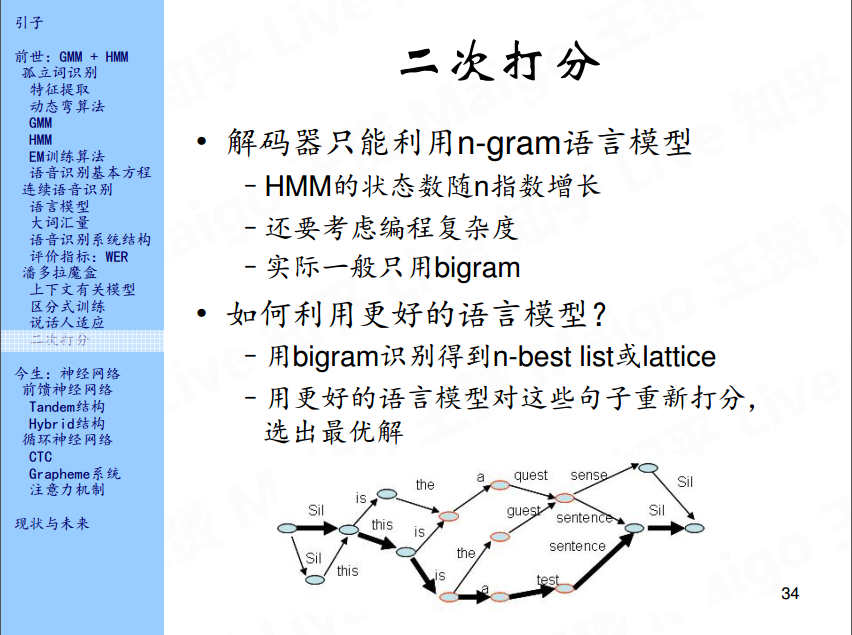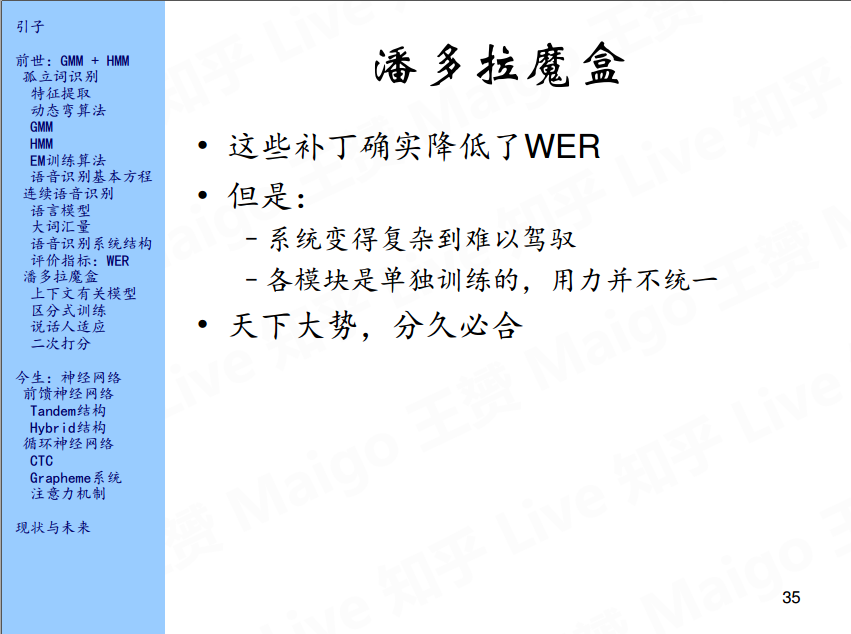# 参考文献

1、语音识别技术的前世今生     https://www.bilibili.com/video/av19158521/

2018-12-04 20:27:38 rickyzhang2008 阅读数 578
• ###### 教你玩转ppt

教你玩转ppt

24139 人正在学习 去看看 刘青

## 算法的提出#

DTW的提出是为了解决或尽量解决在语音识别当中的孤立词识别不正确的问题。该问题简单描述为：在识别阶段，将输入语音的特征矢量时间序列依次与模板库中的每个模板进行相似度比较，最后将相似度最高者作为识别结果输出。但是，由于语音信号具有相当大的随机性，即使是同一个人在不同时刻所讲的同一句话、发的同一个音，也不可能具有完全相同的时间长度。而在进行模板匹配时，这些时间长度的变化会影响测度的估计，从而降低识别率。对此，日本学者 板仓（Itakura）将动态规划（DP）算法的概念用于解决孤立词识别时的说话速度不均匀的难题，提出了著名的动态时间规整算法或称动态时间伸缩算法（DTW）。

## 算法的内容#

DTW的目标是从不同时间跨度的两个数据求出它们之间的最小总累计距离，所以首先我们要找出输入矢量和参考矢量之间的对应关系，从而根据对应的矢量来求出模板之间的最小累计距离。在求累计距离的每一步中，需要满足以下条件的规整函数：

• 边界条件

w(1) = 1，w(N) = M （3-1）

即规整函数起点为（1,1），终点为（N，M），

• 连续条件

w(n + 1) = w(n) + 0/1/2，如果 w(n) <> w(n - 1) 成立 （3-2）

w(n + 1) = w(n) + 1/2，如果 w(n) == w(n - 1) 成立 （3-3）

Tip：

• 式（3-2）意思是 w() 的当前值和前一个 w() 值不相等，说明已经加过 1 或 2 ，则 w(n + 1) 的加上的值可为 0；式（3-3）则刚刚相反，w(n + 1) 的加上的值不能为 0，因为 w(n) 已经使用过 0；本质上，规整函数限制了最小累加距离的路径走向。0/1/2代表了路径走的步数，要么是当前矢量本身，要么是当前矢量的前一列的步数为 1和 2 的矢量（或点）。
• n 的值与 N 相对应

DTW(n,m) = d((n,m)) + min { DTW(n - 1, m) * g(n - 1, m)，DTW(n - 1, m - 1), DTW(n - 1, m - 2)} （3-4）

g(n - 1,m) = 1，如果 w(n - 1) <> w(n - 2) （3-5）

g(n - 1,m) = ∞，如果w(n - 1) == w(n - 2) （3-6）

Tip：这里的只能取前一列的矢量（点）的数据

## 优点和注意点#

### 时间复杂度#

DTW算法的优势在于：它解决了数据长度不同的两数据序列的差异度表示方式。从计算的角度来说，式（3-4）可看出，横轴每向前增加一步，仅参考前一列的累计距离，所以在计算时只保留前一列的累计距离即可，不必保留所有数据。这样可以降低算法的时间复杂度；其原理在于，一个参考数据只与其距离相近的数据会比较相似，距离过远的数据关系不大，所以就没必要计算参考数据与对比数据的所有距离，而DTW算法本身就是在矩阵中运算的，其一般的计算点关系如图### 数据点约束### 计算例子#

d1 = {1,3,3,5,2}，d2 = {0,2,3,6,4,1}

DTW(1,1) = 1 + 0 = 1;
DTW(1,2) = 1 + min {DTW(0,2), DTW(0,1), DTW(0,0)} = 1 + 0 = 1;
DTW(1,3) = 2 + min {DTW(0,3), DTW(0,2), DTW(0,1)} = 2 + 0 = 2;
DTW(1,4) = 5 + min {DTW(0,4), DTW(0,3), DTW(0,2)} = 5 + 0 = 5;
DTW(1,5) = 3 + min {DTW(0,5), DTW(0,4), DTW(0,3)} = 3 + 0 = 3;
DTW(1,6) = 0 + min {DTW(0,6), DTW(0,5), DTW(0,4)} = 0 + 0 = 0;

DTW(2,1) = 3 + min {DTW(1,1), DTW(1,0), DTW(1,-1)} = 3 + 1 = 4;
DTW(2,2) = 1 + min {DTW(1,2), DTW(1,1), DTW(1,0)} = 1 + 1 = 2;
DTW(2,3) = 0 + min {DTW(1,3), DTW(1,2), DTW(1,1)} = 0 + 1 = 1;
DTW(2,4) = 3 + min {DTW(1,4), DTW(1,3), DTW(1,2)} = 3 + 1 = 4;
DTW(2,5) = 1 + min {DTW(1,5), DTW(1,4), DTW(1,3)} = 1 + 2 = 3;
DTW(2,6) = 2 + min {DTW(1,6), DTW(1,5), DTW(1,4)} = 2 + 0 = 2;（这列符合w(n-1) == w(n-2)）

DTW(3,1) = 3 + min {DTW(2,1), DTW(2,0), DTW(2,-1)} = 3 + 4 = 7;
DTW(3,2) = 1 + min {DTW(2,2), DTW(2,1), DTW(2,0)} = 1 + 2 = 3;
DTW(3,3) = 0 + min {DTW(2,3), DTW(2,2), DTW(2,1)} = 0 + 1 = 1;（这列符合w(n-1) == w(n-2)）
DTW(3,4) = 3 + min {DTW(2,4), DTW(2,3), DTW(2,2)} = 3 + 1 = 4;
DTW(3,5) = 1 + min {DTW(2,5), DTW(2,4), DTW(2,3)} = 1 + 1 = 2;
DTW(3,6) = 2 + min {DTW(2,6)∞, DTW(2,5), DTW(2,4)} = 2 + 3 = 5;

DTW(4,1) = 5 + min {DTW(3,1), DTW(3,0), DTW(3,-1)} = 5 + 7 = 12;（这列符合w(n-1) == w(n-2)）
DTW(4,2) = 3 + min {DTW(3,2), DTW(3,1), DTW(3,0)} = 3 + 3 = 6;（这列符合w(n-1) == w(n-2)）
DTW(4,3) = 2 + min {DTW(3,3)
∞, DTW(3,2), DTW(3,1)} = 2 + 3 = 5;
DTW(4,4) = 1 + min {DTW(3,4), DTW(3,3), DTW(3,2)} = 1 + 1 = 2;
DTW(4,5) = 1 + min {DTW(3,5), DTW(3,4), DTW(3,3)} = 1 + 1 = 2;
DTW(4,6) = 4 + min {DTW(3,6), DTW(3,5), DTW(3,4)} = 4 + 2 = 6;

DTW(5,1) = 2 + min {DTW(4,1), DTW(4,0), DTW(4,-1)} = 2 + ∞ = ∞ ;
DTW(5,2) = 0 + min {DTW(4,2), DTW(4,1), DTW(4,0)} = 0 + 12 = 12;
DTW(5,3) = 1 + min {DTW(4,3), DTW(4,2), DTW(4,1)} = 1 + 5 = 6;
DTW(5,4) = 4 + min {DTW(4,4), DTW(4,3), DTW(4,2)} = 4 + 2 = 6;（这列符合w(n-1) == w(n-2)）
DTW(5,5) = 2 + min {DTW(4,5), DTW(4,4), DTW(4,3)} = 2 + 2 = 4;（这列符合w(n-1) == w(n-2)）
DTW(5,6) = 1 + min {DTW(4,6), DTW(4,5), DTW(4,4)} = 1 + 2 = 3;DTW(5,6) 就是最终的累计最短距离，也就是两个数据的差异度表示

2019-09-08 21:58:23 david_tym 阅读数 726
• ###### 教你玩转ppt

教你玩转ppt

24139 人正在学习 去看看 刘青

1，第一阶段

2，第二阶段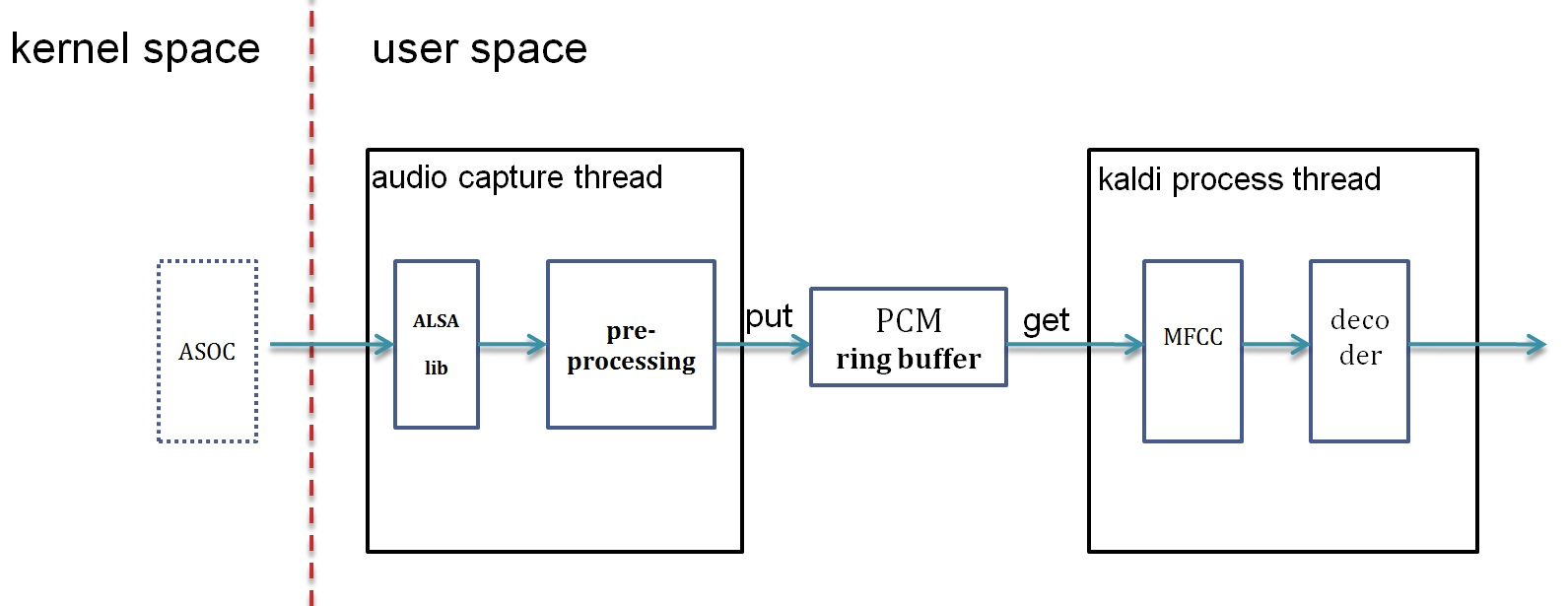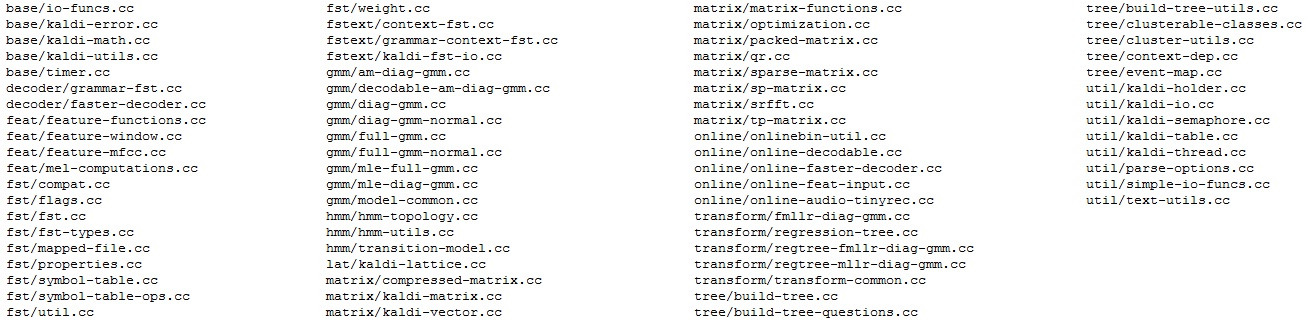3，第三阶段

2020-02-12 18:07:05 weixin_44532659 阅读数 181
• ###### 教你玩转ppt

教你玩转ppt

24139 人正在学习 去看看 刘青

https://pan.baidu.com/s/1C7jgHUH_wuGXKmnvid2EYw